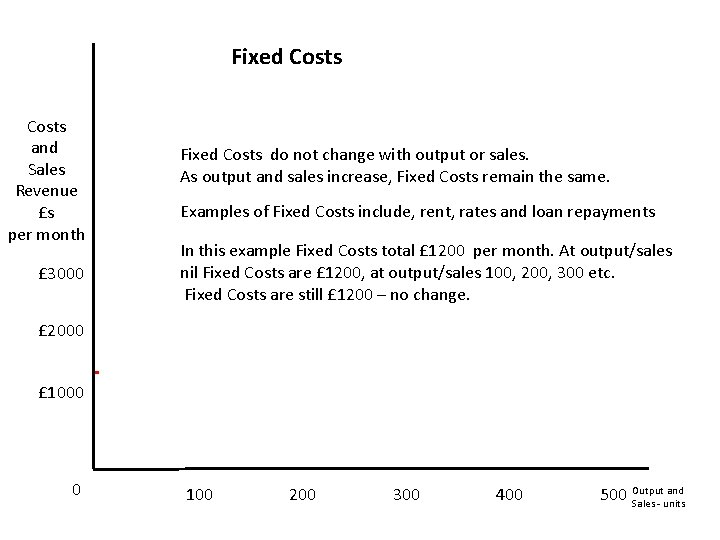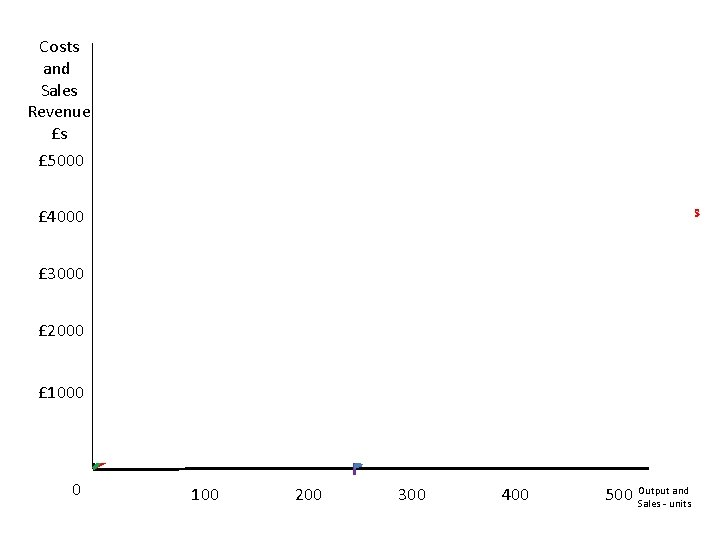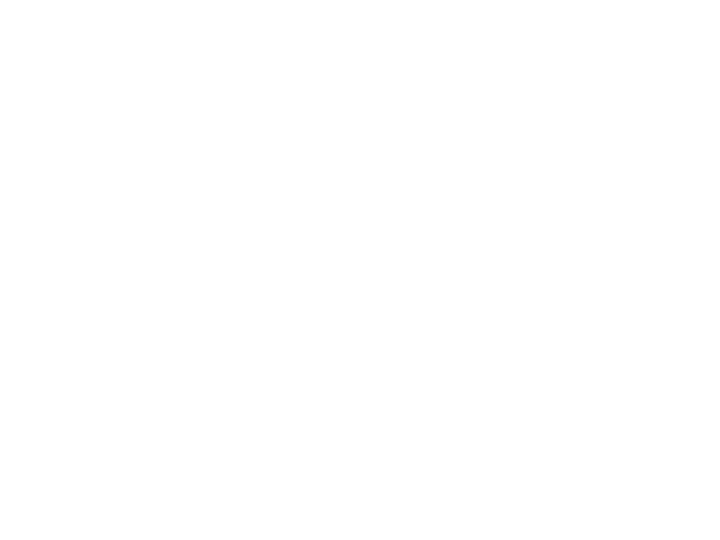# Break Even Diagrams Break Even point is when

• Slides: 10Break Even Diagrams • Break Even point is when a firms total costs are the same as revenue • At break even point the firm is making no profit, and is also making no loss. • At output/sales levels less than break even point the firm is making a loss • At output/sales levels higher than break even point the firm is making a profitThe Break Even Diagram Costs and Sales Revenue £s per month On a break even diagram the vertical (Y) axis shows costs and revenues £ 3000 £ 2000 The horizontal (X) axis shows output and sales £ 1000 0 100 200 300 400 and 500 Output Sales - unitsFixed Costs and Sales Revenue £s per month £ 3000 Fixed Costs do not change with output or sales. As output and sales increase, Fixed Costs remain the same. Examples of Fixed Costs include, rent, rates and loan repayments In this example Fixed Costs total £ 1200 per month. At output/sales nil Fixed Costs are £ 1200, at output/sales 100, 200, 300 etc. Fixed Costs are still £ 1200 – no change. £ 2000 Fixed Costs £ 1000 0 F 100 200 300 400 and 500 Output Sales - unitsCosts and Sales Revenue £s Variable Costs change with output and sales. As output/sales increase, so do Variable Costs. At output nil, Variable Costs are nil. £ 5000 Variable Costs £ 4000 £ 3000 £ 2000 In this example Variable Costs per unit are £ 10. So if we make and sell 1 unit Variable Costs are £ 10. And if we make 400 Variable If we make 100 VCs total £ 1000 F Costs will be £ 4000 £ 1000 0 100 200 300 400 500 Output and Sales - unitsCosts and Sales Revenue £s Total Costs are Fixed Costs plus Variable Costs At Output nil, we have no Variable Costs, just Fixed Costs. So Total Costs are £ 1200. £ 5000 £ 4000 Variable Costs £ 3000 £ 2000 But at higher levels of output/sales, we have Both Fixed and Variable costs. So at output 400, Total Costs = Fixed Costs £ 1200 plus Variable Costs £ 4000 (400 x £ 10) = £ 5200 Fixed Costs £ 1000 0 100 200 300 400 and 500 Output Sales - unitsCosts and Sales Revenue £s £ 5000 Revenue The Revenue line shows us different levels of revenue from different levels of sales. In this case we sell each unit for £ 15 At sales nil then revenue will be nil, but if we sell one then revenue will be £ 15, sell 100 £ 1500. £ 4000 £ 3000 And if we sell 300 revenue will be 300 x £ 15 = £ 4, 500 £ 2000 £ 1000 0 100 200 300 400 and 500 Output Sales - unitsIf we put the costs and revenue Costs lines together we have the complete and Break Even diagram Sales Revenue £s £ 5000 Break Even Point £ 4000 Total Costs Revenue Where Total Costs = Revenue Variable Costs F £ 3000 £ 2000 Fixed Costs £ 1000 Break Even Output 240 units 0 100 200 300 400 and 500 Output Sales - unitsCosts and Sales Revenue £s £ 5000 Profit and Loss Total Costs Revenue Area of profit Break Even Point Variable Costs £ 4000 £ 3000 Area of loss £ 2000 Fixed Costs £ 1000 Break Even Output 0 100 200 300 400 and 500 Output Sales - units